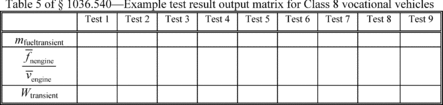# Title 40

## SECTION 1036.540

### 1036.540 Determining cycle-average engine fuel maps.

§ 1036.540 Determining cycle-average engine fuel maps.

(a) Overview. This section describes how to determine an engine's cycle-average fuel maps for model year 2021 and later vehicles with transient cycles. This may also apply for highway cruise cycles as described in § 1036.510. Vehicle manufacturers may need one or both of these to demonstrate compliance with emission standards under 40 CFR part 1037. Generating cycle-average engine fuel maps consists of the following steps:

(1) Determine the engine's torque maps as described in § 1036.510(a).

(2) Determine the engine's steady-state fuel map and fuel consumption at idle as described in § 1036.535.

(3) Simulate several different vehicle configurations using GEM (see 40 CFR 1037.520) to create new engine duty cycles, as described in paragraph (c) of this section. The transient vehicle duty cycles for this simulation are in 40 CFR part 1037, Appendix I; the highway cruise cycles with grade are in 40 CFR part 1037, Appendix IV. Note that GEM simulation relies on vehicle service classes as described in 40 CFR 1037.140.

(4) Test the engines using the new duty cycles to determine fuel consumption, cycle work, and average vehicle speed as described in paragraph (d) of this section and establish GEM inputs for those parameters for further vehicle simulations as described in paragraph (e) of this section.

(b) General test provisions. The following provisions apply for testing under this section:

(1) To perform fuel mapping under this section for hybrid engines, make sure the engine and its hybrid features are appropriately configured to represent the hybrid features in your testing.

(2) Measure NOX emissions for each specified sampling period in grams. You may perform these measurements using a NOX emission-measurement system that meets the requirements of 40 CFR part 1065, subpart J. Include these measured NOX values any time you report to us your fuel consumption values from testing under this section. If a system malfunction prevents you from measuring NOX emissions during a test under this section but the test otherwise gives valid results, you may consider this a valid test and omit the NOX emission measurements; however, we may require you to repeat the test if we determine that you inappropriately voided the test with respect to NOX emission measurement.

(3) This section uses engine parameters and variables that are consistent with 40 CFR part 1065.

(c) Create engine cycles. Use GEM to simulate several different vehicle configurations to create transient and highway cruise engine cycles corresponding to each vehicle configuration, as follows:

(1) Set up GEM to simulate vehicle operation based on your engine's torque maps, steady-state fuel maps, and fuel consumption at idle as described in paragraph (a)(1) and (2) of this section.

(2) Set up GEM with transmission gear ratios for different vehicle service classes and vehicle duty cycles as described in Table 1 of this section. These values are based on automatic or automated manual transmissions, but they apply for all transmission types.

Table 1 of § 1036.540 - Assigned Transmission Gear Ratios

Gear number Light HDV and
medium HDV
Tractors and
heavy HDV,
transient cycle
Tractors and
heavy HDV,
highway cruise
cycle
1 3.10 3.51 12.8
2 1.81 1.91 9.25
3 1.41 1.43 6.76
4 1.00 1.00 4.90
5 0.71 0.74 3.58
6 0.61 0.64 2.61
7 1.89
8 1.38
9 1.00
10 0.73

(3) Run GEM for each simulated vehicle configuration as follows: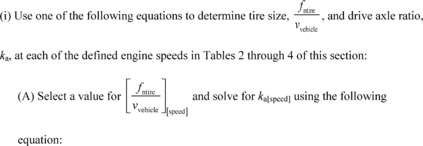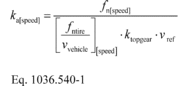Where: fn[speed] = engine's angular speed as determined in paragraph (c)(3)(ii) or (iii) of this section. ktopgear = transmission gear ratio in the highest available gear from Table 4 of this section (for powertrain testing use actual top gear ratio). vref = reference speed. Use 65 mi/hr for the transient cycle and the 65 mi/hr highway cruise cycle, and use 55 mi/hr for the 55 mi/hr highway cruise cycle.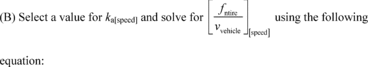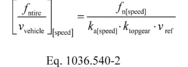Example:

This example is for a vocational Light HDV or vocational Medium HDV with a 6-speed automatic transmission at B speed (Test 3 or 4 in Table 2 of this section).

fnrefB = 1870 r/min = 31.17 r/s kaB = 4.0 ktopgear = 0.61 vref = 65 mi/hr = 29.06 m/s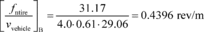(ii) Test at least eight different vehicle configurations for engines that will be installed in vocational Light HDV or vocational Medium HDV. If the engine will also be installed in vocational Heavy HDV, use good engineering judgment to select at least nine test configurations that best represent the range of vehicles. For example, if your engines will be installed in vocational Medium HDV and vocational Heavy HDV, you might select Tests 1 through 6 of Table 2 of this section to represent Class 7 vehicles and Tests 3, 6, and 9 of Table 3 of this section to represent Class 8 vehicles. You may test your engine using additional vehicle configurations with different ka and Crr values to represent a wider range of in-use vehicle configurations. Set CdA to 5.4 for all test configurations. For powertrain testing, set Mrotating to 340 kg and Effaxle to 0.955 for all test configurations.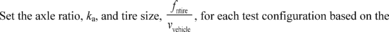corresponding designated engine speed (A, B, C, or fntest) at 65 mi/hr for the transient cycle and the 65 mi/hr highway cruise cycle, and at 55 mi/hr for the 55 mi/hr highway cruise cycle. These engine speeds apply equally for engines subject to spark-ignition standards. Use the following settings specific to each vehicle configuration: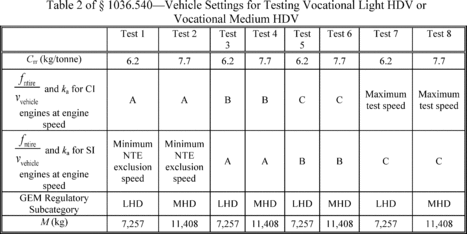(iii) Test nine different vehicle configurations for engines that will be installed in vocational Heavy HDV and for tractors that are not heavy-haul tractors. Test over six different test configurations for heavy-haul tractors. You may test your engines for additional configurations with different ka, CdA, and Crr values to represent a wider range of in-use vehicle configurations. Set Crr to 6.9 for all nine defined test configurations. For powertrain testing, set Effaxle to 0.955 for all test configurations. Set the axle ratio, ka,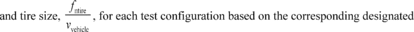engine speed (B, fntest, or the minimum NTE exclusion speed as determined in 40 CFR 86.1370(b)(1)) at 65 mi/hr. Use the settings specific to each test configuration as shown in Table 3 or Table 4 of this section, as appropriate. Engines subject to testing under both Table 3 and Table 4 of this section need not repeat overlapping test configurations, so complete fuel mapping requires testing 12 (not 15) test configurations for those engines. Note that Mrotating is needed for powertrain testing but not for engine testing. Tables 3 and 4 follow: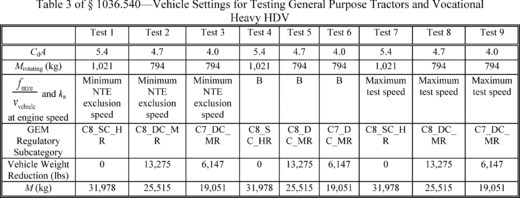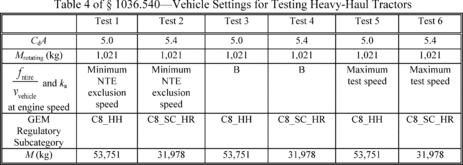(iv) Use the defined values in Tables 1 through 4 of this section to set up GEM with the correct regulatory subcategory and vehicle weight reduction, if applicable, to achieve the target vehicle mass, M, for each test.

(4) Use the GEM output of instantaneous engine speed and engine flywheel torque for each of the vehicle configurations to generate a 10 Hz transient duty cycle corresponding to each vehicle configuration operating over each vehicle duty cycle.

(d) Test the engine with GEM cycles. Test the engine over each of the transient duty cycles generated in paragraph (c) of this section as follows:

(1) Precondition the engine either as described in 40 CFR 1037.510(a)(2)(i) for the transient duty-cycle and 40 CFR 1037.510(a)(2)(ii) for the highway cruise duty cycles using the Test 1 vehicle configuration, and then continue testing the different configurations in the order presented in this section. Measure emissions as described in 40 CFR part 1065; perform cycle validation according to 40 CFR part 1065, subpart F, except as noted in this paragraph (d)(1). If the range of reference speeds is less than 10 percent of the mean reference speed, you need to meet only the standard error of estimate in Table 2 of 40 CFR 1065.514. For purposes of cycle validation, treat points as being at idle if reference speed is at or below declared idle speed. For plug-in hybrid engines, precondition the battery and then complete all back-to-back tests for each test configuration according to 40 CFR 1066.501 before moving to the next test configuration. You may send signals to the engine controller during the test, such as current transmission gear and vehicle speed, if that allows engine operation during the test to better represent in-use operation.

(2) If an infrequent regeneration event occurs during a mapping test interval, invalidate that test interval. Continue operating the vehicle to allow the regeneration event to finish, then repeat engine preconditioning and resume testing at the start of the invalidated test cycle.

(3) For each test, record measurements needed to determine fuel mass using carbon mass balance. Record speed and torque and measure emissions and other inputs as described in 40 CFR 1065.655(c). Manufacturers may instead measure fuel consumption with a fuel flow meter. For hybrid powertrains with no plug-in capability, correct for the net energy change of the energy storage device as described in 40 CFR 1066.501. For plug-in hybrid engines, follow 40 CFR 1066.501 to determine End-of-Test for charge-depleting operation; to do this, you must get our advance approval for a utility factor curve. We will approve your utility factor curve if you can show that you created it from sufficient in-use data of vehicles in the same application as the vehicles in which the PHEV engine will be installed.

(4) Calculate the fuel mass flow rate, mfuel, for each duty cycle using one of the following equations:

(i) Determine fuel-consumption rates using emission measurements from the raw or diluted exhaust, calculate the mass of fuel for each duty cycle, mfuel[cycle], as follows:

(A) For calculations that use continuous measurement of emissions and continuous CO2 from urea, calculate mfuel[cycle] using the following equation: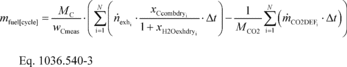Where: MC = molar mass of carbon. wCmeas = carbon mass fraction of fuel (or mixture of test fuels) as determined in 40 CFR 1065.655(d), except that you may not use the default properties in Table 1 of 40 CFR 1065.655 to determine a, b, and wC for liquid fuels. i = an indexing variable that represents one recorded emission value. N = total number of measurements over the duty cycle. n exh = exhaust molar flow rate from which you measured emissions. xCcombdry = amount of carbon from fuel and any injected fluids in the exhaust per mole of dry exhaust as determined in 40 CFR 1065.655(c). xH2Oexhdry = amount of H2O in exhaust per mole of exhaust as determined in 40 CFR 1065.655(c). Δt = 1/frecord. MCO2 = molar mass of carbon dioxide. m CO2DEF = mass emission rate of CO2 resulting from diesel exhaust fluid decomposition over the duty cycle as determined from § 1036.535(b)(10). If your engine does not utilize diesel exhaust fluid for emission control, or if you choose not to perform this correction, set m CO2DEF equal to 0. Example: MC = 12.0107 g/mol wCmeas = 0.867 N = 6680 n exh1= 2.876 mol/s n exh2= 2.224 mol/s xCcombdry1= 2.61·10−3 mol/mol xCcombdry2= 1.91·10−3 mol/mol xH2Oexhdry1= 3.53·10−2 mol/mol xH2Oexhdry2= 3.13·10−2 mol/mol frecord = 10 Hz Δt = 1/10 = 0.1 s MCO2 = 44.0095 g/mol m CO2DEF1 = 0.0726 g/s m CO2DEF2 = 0.0751 g/s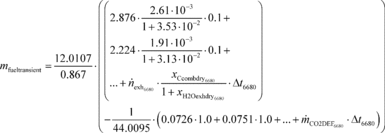Mfueltransient = 1619.6 g

(B) If you measure batch emissions and continuous CO2 from urea, calculate mfuel[cycle] using the following equation: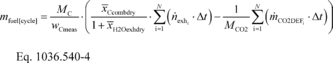(C) If you measure continuous emissions and batch CO2 from urea, calculate mfuel[cycle] using the following equation: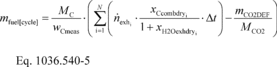(D) If you measure batch emissions and batch CO2 from urea, calculate mfuel[cycle] using the following equation: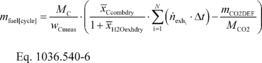(ii) Manufacturers may choose to measure fuel mass flow rate. Calculate the mass of fuel for each duty cycle, mfuel[cycle], as follows: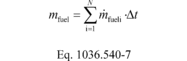Where: i = an indexing variable that represents one recorded value. N = total number of measurements over the duty cycle. For batch fuel mass measurements, set N = 1. m fueli = the fuel mass flow rate, for each point, i, starting from i = 1. Δt = 1/frecord frecord = the data recording frequency. Example: N = 6680 m fuel1 = 1.856 g/s m fuel2 = 1.962 g/s frecord = 10 Hz Δt = 1/10 = 0.1 s mfueltransient = (1.856 + 1.962 + . . . + m fuel6680)·0.1 mfueltransient = 111.95 g

(5) Correct the measured or calculated fuel mass flow rate, mfuel, for each test result to a mass-specific net energy content of a reference fuel as described in § 1036.535(b)(11), replacing with m fuel with mfuel in Eq. 1036.535-3.

(6) For engines designed for plug-in hybrid electric vehicles, the mass of fuel for each cycle, mfuel[cycle], is the utility factor-weighted fuel mass. This is done by calculating mfuel for the full charge-depleting and charge-sustaining portions of the test and weighting the results, using the following equation: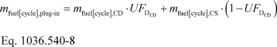Where: mfuel[cycle],CD = total mass of fuel for all the tests in the charge-depleting portion of the test. UFDCD = utility factor fraction at distance DCD as determined by interpolating the approved utility factor curve. mfuel[cycle],CS = total mass of fuel for all the tests in the charge-sustaining portion of the test.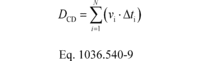Where: v = vehicle velocity at each time step. For tests completed under this section, v is the vehicle velocity in the GEM duty-cycle file. For tests under 40 CFR 1037.550, v is the vehicle velocity as determined by Eq. 1037.550-1. Note that this should include complete and incomplete charge-depleting tests.

(e) Determine GEM inputs. Use the results of engine testing in paragraph (d) of this section to determine the GEM inputs for the transient duty cycle and optionally for each of the highway cruise cycles corresponding to each simulated vehicle configuration as follows:

(1) Your declared fuel mass consumption, mfueltransient. The declared values may be at or above the values calculated in paragraph (d) of this section, as described in § 1036.535(e).

(2) Engine output speed per unit vehicle speed,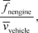by taking the average engine speed measured during the engine test while the vehicle is moving and dividing it by the average vehicle speed provided by GEM. Note that the engine cycle created by GEM has a flag to indicate when the vehicle is moving.

(3) Positive work determined accordering to 40 CFR 1065, Wtransient.

(4) The following table illustrates the GEM data inputs corresponding to the different vehicle configurations: